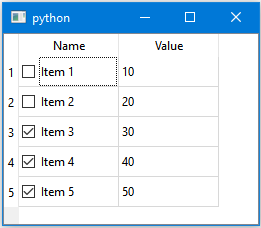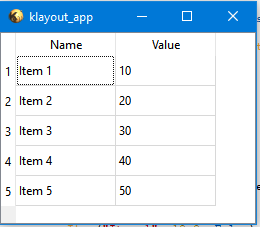# QAbstractTableModel does not draw checkbox for checkable items

Hello,

I am creating table model for my pya based gui and I was able to insert checkbox as widget while using QTableWidget, but after switching to QTableView I don't see checkbox, however, I developed similar application using raw PyQt and checkbox was there.
I am trying to put it into first column and here is my model:

``````class DRCTableModel(pya.QAbstractTableModel):
...
def data(self, index, role=pya.Qt_ItemDataRole.DisplayRole):
if role == pya.Qt_ItemDataRole.DisplayRole.to_i():
if index.column() == 0:
value = self.rules[index.row()].rule
elif index.column() == 1:
value = self.rules[index.row()].value
elif index.column() == 2:
if self.rules[index.row()].status != RuleStatus.UNKNOWN:
value = self.rules[index.row()].status.name
else:
value = ""
elif index.column() == 3:
value = self.rules[index.row()].category
elif index.column() == 4:
value = self.rules[index.row()].description
else:
value = ""
return value
elif role == pya.Qt_ItemDataRole.CheckStateRole.to_i() and index.column() == 0:
return pya.Qt_CheckState.Checked if self.rules[index.row()].is_checked else pya.Qt_CheckState.Unchecked
elif role == pya.Qt_ItemDataRole.BackgroundColorRole.to_i():
if index.column() == 2 and self.rules[index.row()].status == RuleStatus.PASS:
bgColor = pya.QColor(188, 247, 204)
elif index.column() == 2 and self.rules[index.row()].status == RuleStatus.FAIL:
bgColor = pya.QColor(255, 187, 181)
else:
bgColor = pya.QColor(pya.Qt.white)
return bgColor
else:
return pya.QVariant()

def flags(self, index):
fl = pya.QAbstractTableModel.flags(self, index)
if index.column() == 0:
fl |= pya.Qt_ItemFlag.ItemIsEditable | pya.Qt_ItemFlag.ItemIsUserCheckable
return fl

def setData(self, index, value, role=pya.Qt_ItemDataRole.EditRole):
if not index.isValid():
return False
if role == pya.Qt_ItemDataRole.CheckStateRole.to_i():
self.rules[index.row()].is_checked = value
return True
return False
``````

I presented only those methods that important for checkbox column functionality.
Do I need actually to implement my own delegate? Or checkable item functionality does exists in pya?

thank you

• See https://www.klayout.de/forum/discussion/2047/problem-with-qtreewidgetitem-setbackground as example of `CheckState` usage in `QTreeWidget`.

• Hi @EugeneZelenko, thanks for the comment. I am not sure that understand it completely, but it looks like you are referring to changing check state of particular item in your QTreeWidget, am I right?
The point is that this is widget based way and it works for me as well (for QTableWidget) but I am trying switching to model-view architecture and check state in this way can be controlled through model directly. As I said, with raw Qt or PyQt I know how to do that but here checkbox is not plotting. Please, correct me if I misinterpreted your message.

Custom editing elements can be handled through user's delegate class, but for checking items it was always possible to use standard delegate.

• @alexlpn I cannot debug or reproduce that isuee with partial code. Could you paste a full sample that allows me to reproduce the problem?

The reason may be manifold. I'm not using the Qt classes to an extent I have much experience with that myself.

A note of caution: the Qt bindings do not have the quality of PyQt, but I could not use PyQt. The reason is that PyQt is build atop of Python while in KLayout, Python sits atop of the application. That is a difference. Plus there was not PyQt equivalent for Ruby. So KLayout's Qt binding is supposed to provide the necessary API for utility UIs, but C++ to Python transition is tricky and I cannot give warranty that all functionality is properly implemented. The Qt API is simply too huge to give it a thorough testing.

I'd like to debug the issue, but please do not ask me to create a functional sample myself.

Matthias

• Hi @Matthias , here I tried to compile small example (actually two).
This one is raw PyQt5 based:

``````from PyQt5.QtCore import QAbstractTableModel, QModelIndex, Qt, QVariant
from PyQt5.QtWidgets import QApplication, QMainWindow, QTableView
from dataclasses import dataclass, fields
from typing import List

@dataclass
class Item:
name: str
value: float
is_checked: bool

class Model(QAbstractTableModel):
def __init__(self):
super().__init__()
self.items = []

def setItems(self, items: List[Item]):
self.items = items
self.layoutChanged.emit()

def setAllItemsSelected(self, selected: bool = True):
for r in self.items:
r.is_checked = selected
self.layoutChanged.emit()

def rowCount(self, parent=QModelIndex()):
return len(self.items)

def columnCount(self, parent=QModelIndex()):
return len(fields(Item)) - 1

def data(self, index, role=Qt.DisplayRole):
if role == Qt.DisplayRole:
if index.column() == 0:
value = self.items[index.row()].name
elif index.column() == 1:
value = self.items[index.row()].value
else:
value = ""
return value
elif role == Qt.CheckStateRole and index.column() == 0:
return Qt.Checked if self.items[index.row()].is_checked else Qt.Unchecked
else:
return QVariant()

if role == Qt.DisplayRole and orientation == Qt.Horizontal:
if section == 0:
value = "Name"
elif section == 1:
value = "Value"
else:
value = ""
return value
elif role == Qt.DisplayRole and orientation == Qt.Vertical:
return section + 1

def flags(self, index):
fl = QAbstractTableModel.flags(self, index)
if index.column() == 0:
fl |= Qt.ItemIsEditable | Qt.ItemIsUserCheckable
return fl

class MainWindow(QMainWindow):
def __init__(self, parent=None):
super().__init__()
table = QTableView(self)
model = Model()
table.setModel(model)
self.setCentralWidget(table)

items = [
Item("Item 1", 10.0, False),
Item("Item 2", 20.0, False),
Item("Item 3", 30.0, True),
Item("Item 4", 40.0, True),
Item("Item 5", 50.0, True),
]

model.setItems(items)

if __name__ == "__main__":
app = QApplication([])
wnd = MainWindow()
wnd.show()
app.exec()
``````

This one is pya based (the difference is in Qt.CheckStateRole -> Qt.CheckStateRole.to_i() and similar roles :

``````from pya import QAbstractTableModel, QModelIndex, Qt, QVariant
from pya import QMainWindow, QTableView
from dataclasses import dataclass, fields
from typing import List

@dataclass
class Item:
name: str
value: float
is_checked: bool

class Model(QAbstractTableModel):
def __init__(self):
super().__init__()
self.items = []

def setItems(self, items: List[Item]):
self.items = items
self.emit_layoutChanged()

def setAllItemsSelected(self, selected: bool = True):
for r in self.items:
r.is_checked = selected
self.emit_layoutChanged()

def rowCount(self, parent=QModelIndex()):
return len(self.items)

def columnCount(self, parent=QModelIndex()):
return len(fields(Item)) - 1

def data(self, index, role=Qt.DisplayRole):
if role == Qt.DisplayRole.to_i():
if index.column() == 0:
value = self.items[index.row()].name
elif index.column() == 1:
value = self.items[index.row()].value
else:
value = ""
return value
elif role == Qt.CheckStateRole.to_i() and index.column() == 0:
return Qt.Checked if self.items[index.row()].is_checked else Qt.Unchecked
else:
return QVariant()

if role == Qt.DisplayRole.to_i() and orientation == Qt.Horizontal:
if section == 0:
value = "Name"
elif section == 1:
value = "Value"
else:
value = ""
return value
elif role == Qt.DisplayRole.to_i() and orientation == Qt.Vertical:
return section + 1

def flags(self, index):
fl = QAbstractTableModel.flags(self, index)
if index.column() == 0:
fl |= Qt.ItemIsEditable | Qt.ItemIsUserCheckable
return fl

class MainWindow(QMainWindow):
def __init__(self, parent=None):
super().__init__()
self.table = QTableView(self)
self.model = Model()
self.table.setModel(self.model)
self.setCentralWidget(self.table)

items = [
Item("Item 1", 10.0, False),
Item("Item 2", 20.0, False),
Item("Item 3", 30.0, True),
Item("Item 4", 40.0, True),
Item("Item 5", 50.0, True),
]

self.model.setItems(items)

if __name__ == "__main__":
wnd = MainWindow()
wnd.show()
``````

Here are the plots of what gives PyQt and pya in comparison:Those checkboxes I am talking about. Usually when I am flagging item as Qt.ItemIsUserCheckable this delegate will be plotted automatically.
I hope this highlights the issue.

• edited April 2022

Thanks, a lot for that nice test case.

I could debug the problem and fix it by using

``````        elif role == Qt.CheckStateRole.to_i() and index.column() == 0:
return Qt.Checked.to_i() if self.items[index.row()].is_checked else Qt.Unchecked.to_i()
``````

(note the `to_i()` in the return statement).

Here is my explanation: KLayout treats enums as typed objects. So if you have `Qt.CheckStateRole` it is actually a constant of type `Qt::ItemDataRole`.

That usually works nicely, but for the `QAbstractItemModel`, for some reason the role is declared as "int" in C++. Hence for the comparison you need to convert to int with `to_i`. Maybe that can be simplified if I implement some "enum == int" compare. Until then you'll need `to_i`.

The same thing happens on the return side. The `QVariant` binding does not support "enum type to int" automatic conversion, but the C++ API requires an integer value. So again, you'll need to convert to int again. Maybe it's also possible to rectify that so eventually code is more compatible with PyQt5. Right now, I suggest the "to_i" workaround.

Kind regards,

Matthias

• I have issue a PR which should enable enum-to-int conversion in both directions (https://github.com/KLayout/klayout/pull/1055). This should remove the need for adding to_i. It's a too deep change to go into a minor release, but I'll include it in the next major one.

• Matthias, this is great, thanks a lot!